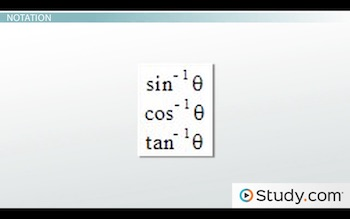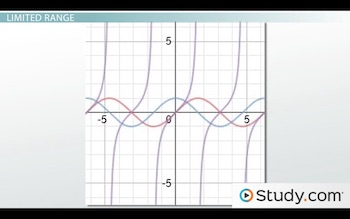# Properties of Inverse Trigonometric Functions

Lesson Transcript
Instructor: Yuanxin (Amy) Yang Alcocer

Amy has a master's degree in secondary education and has taught math at a public charter high school.

After watching this video lesson, you will understand why our inverse trigonometric functions have a limited range. Learn what this does. Also learn the notation for these functions.

## Inverse Trigonometric Functions

In addition to the trigonometric functions that we are familiar with at this point such as sine, cosine, and tangent, we also have what are called the inverse trigonometric functions. It is these functions that we will be talking about in this video lesson. What exactly are they? They are the inverse functions of our trig functions.

However, in trigonometry, the inverse function here is not 1 divided by the function. This inverse function allows you to solve for the argument. For example, if you have the problem sin x = 1, we can solve the problem by multiplying both sides by the inverse sine function. The inverse sine function cancels the sine function on the left side and we are left with x = sin^-1 (1). Evaluating the right side allows us to find the angle of the sine function that fits the problem.

An error occurred trying to load this video.

Try refreshing the page, or contact customer support.

Coming up next: Solving Trigonometric Equations with Restricted Domains

### You're on a roll. Keep up the good work!

Replay
Your next lesson will play in 10 seconds
• 0:01 Inverse Trigonometric…
• 1:00 Notation
• 2:08 Limited Range
• 4:52 Graphs
• 5:40 Lesson Summary
Save Save

Want to watch this again later?

Log in or sign up to add this lesson to a Custom Course.

Timeline
Autoplay
Autoplay
Speed Speed

## Notation

As you have just seen, the notation for these inverse trigonometric functions is unique. We use an exponent of -1 to let us know that we are dealing with the inverse trig function. We can write our inverse trig functions like this:This is the notation that you will see most often in textbooks and various trig problems. And this is most likely the notation you will use when writing out your problems. But, in trigonometry, we also have formal names for these functions. We call the inverse sine function the arcsine function, the inverse cosine function the arccosine function, and the inverse tangent function the arctangent function. While you will see the first notation more often in problems, you will come across these formal names in math discussions. So, it's good to know both. It's easy to remember these names if you link the arc with the -1 exponent. All the inverse trigonometric functions begin with the prefix arc- followed by the name of the trig function that we already know.

## Limited Range

Now, think back to the regular trig functions of sine, cosine, and tangent. Do you remember their graphs?The red line is the graph of the sine function, the blue line the graph of the cosine function and the purple line is the graph of the tangent function. What interesting thing do you notice about these graphs?

All of these graphs repeat every so often. The tangent function repeats every pi spaces while the sine and cosine functions repeat every 2pi spaces. Each time the function repeats, we get the same output answer. Because these functions repeat, we have to limit the range, or output values, of our inverse trig functions. Otherwise, we would get different answers each time.

By limiting the range of our inverse function, we find the principal or primary value of our inverse function. This is what is going inside your calculator whenever you perform an inverse trig function. It gives you an answer within the accepted range. If we didn't limit our range, our calculator wouldn't know which answer to give you since the answers repeat every 2pi for the sine and cosine functions and pi for the tangent function. The following are the accepted limited ranges for our inverse trigonometric functions:

Inverse function Range
y = arcsin x -pi/2 <= y <= pi/2
y = arccos x 0 <= y <= pi
y = arctan x -pi/2 < y < pi/2

These ranges don't exactly correspond to how our regular trig functions repeat. This time, we have the inverse cosine function that is limited between 0 and pi. The inverse sine function is limited to between -pi/2 and pi/2 including those points. The inverse tangent function has the same limited range as the inverse sine except the two points of -pi/2 and pi/2 are not included.

To unlock this lesson you must be a Study.com Member.
Create your account

### Register to view this lesson

Are you a student or a teacher?

### Unlock Your Education

#### See for yourself why 30 million people use Study.com

##### Become a Study.com member and start learning now.
Back
What teachers are saying about Study.com
Create an account to start this course today
Used by over 30 million students worldwide